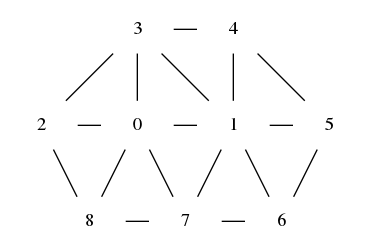# Mesh¶

Represent explicit surfaces on the device with triangle mesh. This was designed for close surfaces.

## Internal structure¶

A mirheo::Mesh is composed of an array of vertices (it contains the coordinates of each vertex) and a list of faces. Each entry of the faces is composed of three indices that correspond to the vertices in the corresponding triangle.

A mirheo::MembraneMesh contains also adjacent information. This is a mapping from one vertex index to the indices of all adjacent vertices (that share an edge with the input vertex).

Example: In the following mesh, suppose that the maximum degree is maxDegree = 7, the adjacent lists have the entries:

1 7 8 2 3 * * 0 3 4 5 6 7 * ...


The first part is the ordered list of adjacent vertices of vertex 0 (the * indicates that the entry will not be used). The second part corresponds to vertex 1. The first entry in each list is arbitrary, only the order is important. The list of adjacent vertices of vertex i starts at i * maxDegree.## API¶

### Host classes¶

class Mesh

A triangle mesh structure.

The topology is represented by a list of faces (three vertex indices per face).

Subclassed by mirheo::MembraneMesh

Public Functions

Mesh()

Default constructor. no vertex and faces.

Mesh(const std::string &fileName)

Construct a Mesh from a off file.

Parameters
• fileName: The name of the file (contains the extension).

Mesh(const std::tuple<std::vector<real3>, std::vector<int3>> &mesh)

Construct a Mesh from a list of vertices and faces.

Mesh(const std::vector<real3> &vertices, const std::vector<int3> &faces)

Construct a Mesh from a list of vertices and faces.

Mesh(Mesh&&)

move constructor

Mesh &operator=(Mesh&&)

move assignment operator

int getNtriangles() const

Return
the number of faces

int getNvertices() const

Return
the number of vertices

int getMaxDegree() const

Return
the maximum valence of all vertices

const PinnedBuffer<real4> &getVertices() const

Return
the list of vertices

const PinnedBuffer<int3> &getFaces() const

Return
the list of faces

class MembraneMesh : public mirheo::Mesh

A triangle mesh with face connectivity, adjacent vertices and geometric precomputed values.

This class was designed to assist MembraneInteraction.

A stress-free state can be associated to the mesh. The precomputed geometric quantities that are stored in the object are computed from the stress free state.

Additionally to the list of faces (

See
Mesh), this class contains a list of adjacent vertices for each vertex. The list is stored in a single array, each vertex having a contiguous chunk of length maxDegree. See developer docs for more information.

Public Functions

MembraneMesh()

construct an empty mesh

MembraneMesh(const std::string &initialMesh)

Construct a MembraneMesh from an off file.

Note
The stress free state will be the one given by initialMesh
Parameters
• initialMesh: File (in off format) that contains the mesh information.

MembraneMesh(const std::string &initialMesh, const std::string &stressFreeMesh)

Construct a MembraneMesh from an off file.

Note
initialMesh and stressFreeMesh must have the same topology.
Parameters
• initialMesh: File (in off format) that contains the mesh information.
• stressFreeMesh: File (in off format) that contains the stress free state of the mesh.

MembraneMesh(const std::vector<real3> &vertices, const std::vector<int3> &faces)

Construct a MembraneMesh from a list of vertices and faces.

Note
The stress free state is the same as the current mesh.
Parameters
• vertices: The vertex coordinates of the mesh
• faces: List of faces that contains the vertex indices.

MembraneMesh(const std::vector<real3> &vertices, const std::vector<real3> &stressFreeVertices, const std::vector<int3> &faces)

Construct a MembraneMesh from a list of vertices and faces.

Parameters
• vertices: The vertex coordinates of the mesh
• stressFreeVertices: The vertex coordinates that represent the stress free state.
• faces: List of faces that contains the vertex indices.

MembraneMesh(MembraneMesh&&)

move constructor

MembraneMesh &operator=(MembraneMesh&&)

move assignment operator

const PinnedBuffer<int> &getAdjacents() const

Return
The adjacency list of each vertex

const PinnedBuffer<int> &getDegrees() const

Return
The degree of each vertex

class MeshDistinctEdgeSets

Stores sets of edges that share the same colors as computed by computeEdgeColors().

This allows to work on edges in parallel with no race conditions.

Public Functions

MeshDistinctEdgeSets(const MembraneMesh *mesh)

Construct a MeshDistinctEdgeSets.

Parameters
• mesh: The input mesh with adjacency lists.

int numColors() const

Return
the number of colors in the associated mesh.

const PinnedBuffer<int2> &edgeSet(int color) const

Return
The list of edges (vertex indices pairs) that have the given color.

### Views¶

struct MeshView

A device-compatible structure that represents a triangle mesh topology (.

See
Mesh)

Subclassed by mirheo::MembraneMeshView

Public Functions

MeshView(const Mesh *m)

Construct a MeshView from a Mesh.

Public Members

int nvertices

number of vertices

int ntriangles

number of faces

int3 *triangles

list of faces

struct MembraneMeshView : public mirheo::MeshView

A device-compatible structure that represents a data stored in a MembraneMesh additionally to its topology.

Public Functions

MembraneMeshView(const MembraneMesh *m)

Construct a MembraneMeshView from a MembraneMesh object.

Public Members

int maxDegree

maximum degree of all vertices

int *adjacent

int *degrees

degree of each vertex

real *initialLengths

lengths of edges in the stress-free state

real *initialAreas

areas of each face in the stress-free state

real *initialDotProducts

do products between adjacent edges in the stress-free state